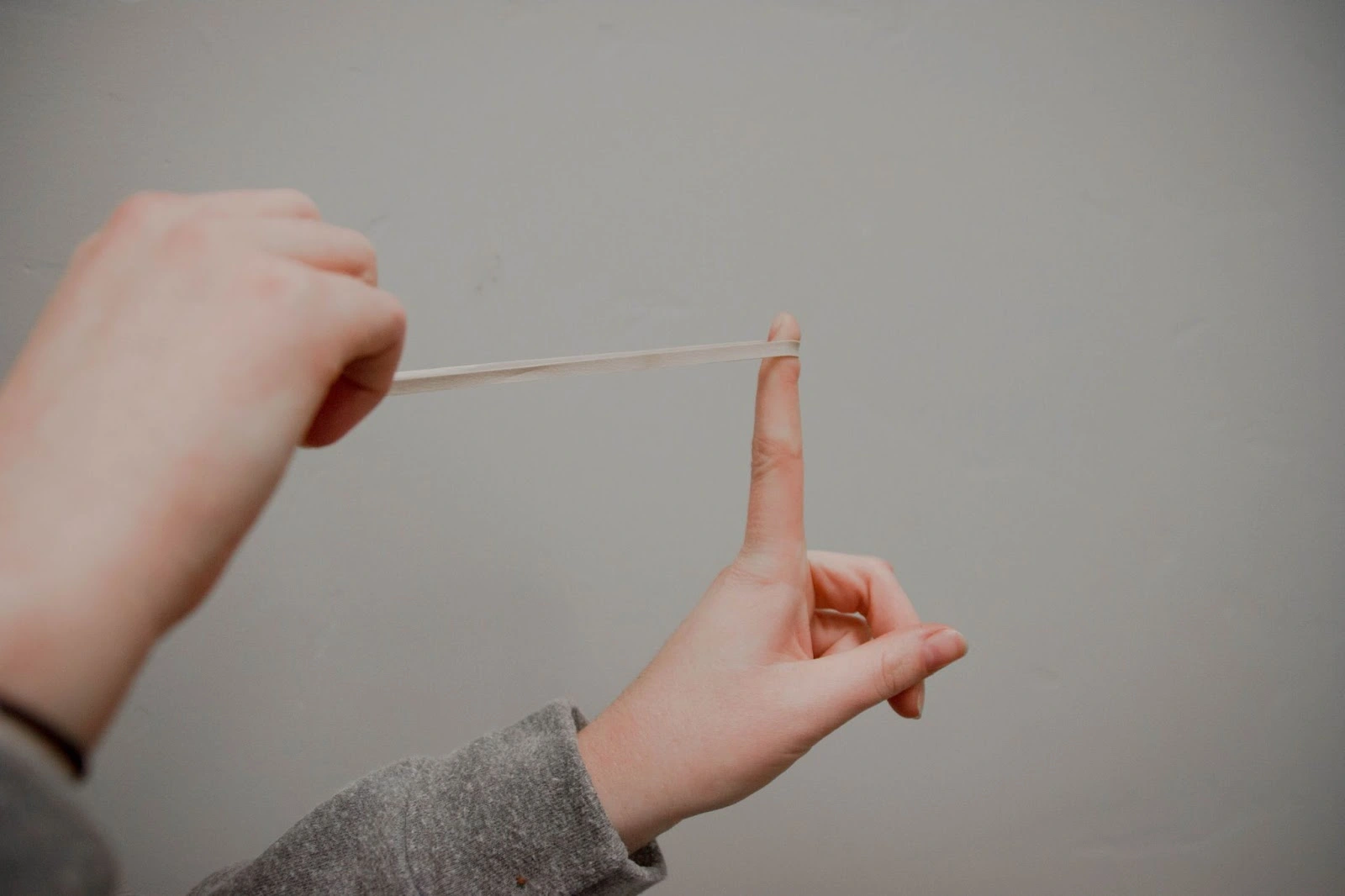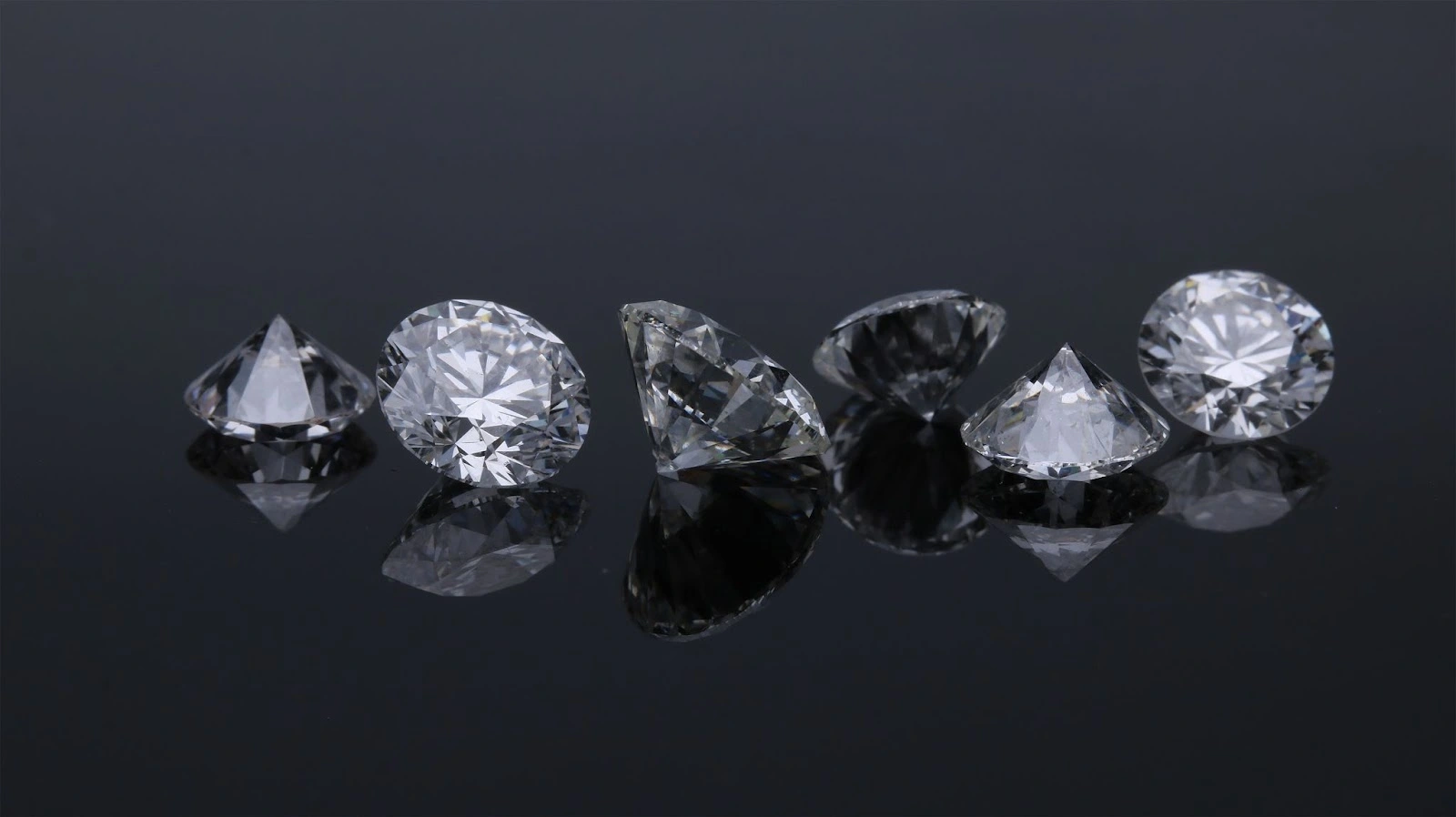Study S1 Science Science - Exploring Diversity of Matter by its Physical Properties - Geniebook# Exploring Diversity Of Matter By Its Physical Properties

In this article, the following are the objectives that will be discussed.

• Understand what physical properties are.
• Understand units of measurement.
• State the SI units of the various physical properties.

## Physical Properties Of Materials

The physical properties of a material are qualities that can be detected by using our five senses (e.g. sense of sight, touch, smell, taste and hearing) or a measuring device.

### Physical Properties:

#### 1. Flexibility

######Image Credit: Kenny Eliason - unsplash.com

It is the ability to bend without breaking and to return to its original shape and size after it is bent.

An example of a material with flexibility is the rubber band.

#### 2. Hardness

######Image Credit:  Edgar Soto - unsplash.com

It is the ability to withstand scratches.

A material can cut or scratch a material that is less hard than itself but not a material that is harder.

The diamond is the hardest material in the world. It cannot be scratched and cut by any other material. A diamond can only be cut by another diamond.

#### 3. Melting Point

The melting point of a material is the temperature at which it changes its state from solid to liquid.

For example: the melting point of water is 0°C.

#### 4. Boiling Point

The boiling point of a material is the temperature at which it changes its state from liquid to gas.

For example: the boiling point of water is 100°C.

Let’s understand this with the help of some examples:

#### 5. Strength

######Image credit: Grahame Jenkins - unsplash.com

It is the ability to support heavy loads without tearing or breaking.

Strong materials could be flexible but not all flexible materials are strong materials.

A car frame is made up of metal because it is a strong material.

#### 6. Thermal Conductivity

It is a measure of how readily heat passes through a material.

Heat conductors allow heat to pass through them easily.

Heat insulators or poor heat conductors do not allow heat to pass through them easily.

Metals are generally good conductors of heat. Rubber, glass, plastic and other non-metals are poor conductors of heat.

For example: Rubber is a heat insulator. It allows heat to pass through them but at a very slow rate.

#### 7. Electrical Conductivity

It is a measure of how readily electricity passes through a material.

Electrical conductors allow electricity to pass through them easily. All metals are electrical conductors.

Electrical insulators do not allow electricity to pass through them.

#### 8. Density

It is the quantity of matter packed into a unit volume of the material.

\begin{align} \text{Density} = \frac{\text{Mass}}{\text{Volume}} \end{align}

A material that is denser will sink in a liquid which is less dense than them.

A material that is less dense will float in a liquid that is denser than them.

## Units Of Measurement

Measurements are expressed in two parts:

1. numeric value; and
2. unit.

For example: The measurement of the length is $$\text{12 cm}$$. So, $$\text{12}$$ is the numeric value and $$\text{cm}$$ is unit.

## SI Units

International System of Units, also known as SI unit, is established to ensure common standards in the measurements.

 Physical Quantity SI Unit Symbol length metre m mass kilogram kg temperatute Kelvin K time second s

### Mass

Mass is a measure of the quantity of matter in a body.

The SI unit of mass is kilogram ($$\text{kg}$$).

The electronic balance, triple-beam balance and four-beam pan balance are instruments used to measure mass.

### Length

Length is the distance between two specified points.

The SI unit of length is metre ($$\text{m}$$).

The measuring tape, metre rule and Vernier calliper are instruments used to measure length.

### Volume

Volume is the amount of space an object occupies.

The SI unit of volume is cubic metre ($$\text{m}^3$$).

The measuring cylinder is often used to measure volumes of liquids.

## Revision Questions

Here are some questions for us to look into on the objectives of this article.

Question 1:

Which of the following is not one of the physical properties of materials?

1. flexibility
2. hardness
3. happiness
4. strength

Solution:

(C) happiness

Explanation:

The physical properties of a material are qualities that can be detected by using our five senses or a measuring device.Happiness cannot be detected by using our five senses nor by a measuring device.

Question 2:

Which of the following refers to the ability to support heavy loads without breaking or tearing?

1. flexibility
2. hardness
3. density
4. strength

Solution:

(D) strength

Explanation:

The strength of a material is its ability to support a heavy load without breaking or tearing.

Question 3:

Which of the following refers to the ability to bend without breaking?

1. flexibility
2. hardness
3. density
4. strength

Solution:

(A) flexibility

Explanation:

The flexibility of a material is its ability to bend without breaking and to be able to return to its original size and shape after bending.

Question 4:

Which of the following refers to the ability to withstand scratches?

1. heat conductivity
2. hardness
3. density
4. strength

Solution:

(B) hardness

Explanation:

The hardness of a material is its ability to withstand scratches.

Question 5:

Which of the following is the SI unit of temperature?

1. Celsius
2. Fahrenheit
3. Kelvin

Solution:

(C) Kelvin

Explanation:

The SI unit of temperature is Kelvin (K).

Question 6:

Which of the following is the SI unit of length?

1. kilometres
2. centimetres
3. metres
4. inch

Solution:

(C) metres

Explanation:

The SI unit of length is metres.

Question 7:

Which of the following is the instrument to measure length?

1. electronic balance
2. measuring cylinder
3. Vernier calliper
4. thermometer

Solution:

(C) Vernier calliper

Explanation:

The electronic balance is used to measure mass.

The measuring cylinder is used to measure volume.

The thermometer is used to measure temperature.

Question 8:

Which of the following is the instrument to measure mass?

1. electronic balance
2. measuring cylinder
3. Vernier calliper
4. thermometer

Solution:

(A) electronic balance

Explanation:

The measuring cylinder is used to measure volume.

The Vernier calliper is used to measure length.

The thermometer is used to measure temperature

Continue Learning
Model Of Cells - The Basic Unit Of Life Solutions And Suspensions
Model Of Matter - Atoms And Molecules Exploring The Diversity Of Matter By Its Chemical Composition
Exploring Diversity Of Matter By Its Physical Properties Exploring Diversity Of Matter Using Separation Techniques
Model of Matter - The Particulate Nature Of Matter Ray Model Of Light
Human Digestive SystemPrimaryPrimary 1Primary 2Primary 3Primary 4Primary 5Primary 6SecondarySecondary 1EnglishMathsScience
Model Of Cells - The Basic Unit Of Life
Solutions And Suspensions
Model Of Matter - Atoms And Molecules
Exploring The Diversity Of Matter By Its Chemical Composition
Exploring Diversity Of Matter By Its Physical Properties
Exploring Diversity Of Matter Using Separation Techniques
Model of Matter - The Particulate Nature Of Matter
Ray Model Of Light
Human Digestive SystemSecondary 2Secondary 3Secondary 4